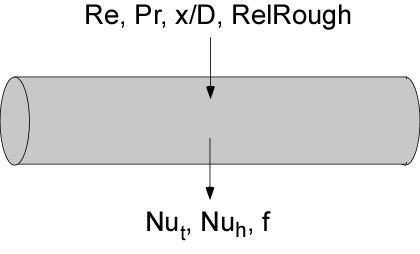Contents - Index

PipeFlow_ND_localProcedure PipeFlow_ND_local(Re, Pr, xoverD, RelRough: Nusselt_T_x, Nusselt_H_x, f_x) returns the local Nusselt numbers assuming constant temperature wall and constant heat flux for a given flow condition a circular tube characterized by Reynolds and Prandtl numbers,axial position/diameter, and relative roughness.  The Procedure also returns the local friction factor for these conditions.   The procedure can be used for developing or fully-developed flow in the laminar, transitional or turbulent flow regimes.

Inputs:

Re - Reynolds number based on pipe diameter and the properties evaluated at the local bulk average temperature

Pr - Prandtl number of the fluid evaluated at the local bulk average temperature

xoverD - ratio of axial position to diameter.

RelRough - the ratio of the dispersions on the wall of the tube to the tube diameter (must be between 0 and 0.05)

Outputs:

Nusselt_T_x - local Nusselt number assuming a constant wall temperature

Nusselt_H_x - local Nusselt number assuming a constant wall heat flux

f_x -  local friction factor [-]

Notes

This procedure  determines the local Nusselt numbers and friction factor by differentiating the average values provided by the PipeFlow_ND procedure.  See PipeFlow_ND for more information.

The Nusselt numbers approach infinity as xoverD goes to 0.  The correlations are not applicable for xoverD ratios less than 0.1.

Two Nusselt numbers are returned.  Nusselt_T corresponds to a constant temperature wall and Nusselt_H corresponds to a constant heat flux.  These two values will be the same for turbulent flow.  For laminar flow, they provide upper and lower bounds on the Nusselt number.

Example

Re=1000

Pr=2

xoverD=1.1

relRough=0

call PipeFlow_ND_local(Re,Pr,xoverD,RelRough: Nusselt_T_x,Nusselt_H_x, f_x)

{Solution:

f_x=0.2156 [-]

Nusselt_T_x=14.03 [-]

Nusselt_H_x=20.05 [-]}